Consider the three displacement vectors = ( 4î − 3ĵ) m, = (3î − 6ĵ) m, and = (−6î + 5ĵ) m. Use the component method to determine the followi

Question

Consider the three displacement vectors = ( 4î − 3ĵ) m, = (3î − 6ĵ) m, and = (−6î + 5ĵ) m. Use the component method to determine the following. (Take the +x direction to be to the right.)

(a) the magnitude and direction of the vector = Darrowbold = A with arrow + B with arrow + C with arrow

magnitude=____ m

direction=____ ° counterclockwise from the +x axis(b) the magnitude and direction of E with arrow = −A with arrow − B with arrow + C with arrow magnitude

in progress 0
4 months 2021-09-05T01:19:38+00:00 1 Answers 16 views 0

(a).The magnitude and direction of the vector is 4.12 m and 284°

(b). The magnitude and direction of the vector is 19.10 m and 313°

Explanation:

Given that,

The  three displacement are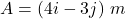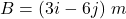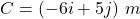We need to calculate the magnitude of the vector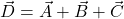Put the value into the formula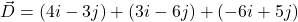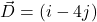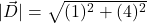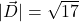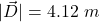We need to calculate the direction of the vector

Using formula of direction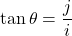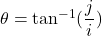Put the value into the formula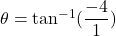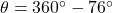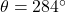(b). We need to calculate the magnitude of the vector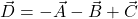Put the value into the formula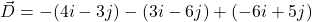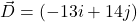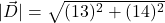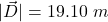We need to calculate the direction of the vector

Using formula of directionPut the value into the formula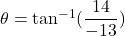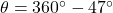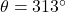Hence, (a).The magnitude and direction of the vector is 4.12 m and 284°

(b). The magnitude and direction of the vector is 19.10 m and 313°